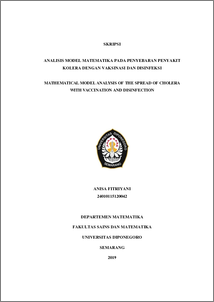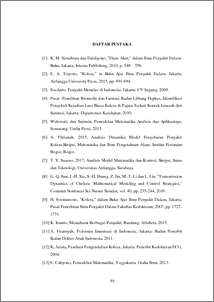# MATHEMATICAL MODEL ANALYSIS OF THE SPREAD OF CHOLERA WITH VACCINATION AND DISINFECTION

Fitriyani, Anisa (2019) MATHEMATICAL MODEL ANALYSIS OF THE SPREAD OF CHOLERA WITH VACCINATION AND DISINFECTION. Undergraduate thesis, UNDIP.Preview
PDF
308KbPDF
Restricted to Repository staff only

1295KbPreview
PDF
186Kb

## Abstract

Cholera is a disease as kind of acute diarrhea caused by bacteria V. Cholerae, which can be spread through the direct contact of humans and contaminated food or water. The spread of cholera can be prevented by vaccination and disinfection. This phenomenon is modeled in the form of first-order non-linear differential equation systems with 4 variables, SIRB . The model has two equilibrium points namely disease-free equilibrium points and endemic equilibrium points. Also obtained are basic reproductive numbers ( R0 ) which are used to determine the local stability at the equilibrium point. Disease-free equilibrium points are locally asymptotically stable if R0 1 , while endemic equilibrium points are locally asymptotically stable if R0 1 . Furthermore, the simulation results obtained R0  0.87 , this indicates that the local asymptotic stable system at the equilibrium point is disease free, whereas in the endemic conditions where R0 1 , an increase in vaccination rates and disinfection rate can reduce the population of susceptible individuals, infected, and bacteria V. Cholerae. Keywords: Cholera, Vaccination, Disinfection, basic reproduction numbers, local stability

Item Type: Thesis (Undergraduate) Q Science > QA Mathematics Faculty of Science and Mathematics > Department of Mathematics 84231 INVALID USER 12 Jun 2022 05:26 12 Jun 2022 05:26

Repository Staff Only: item control page